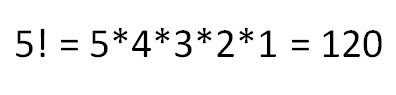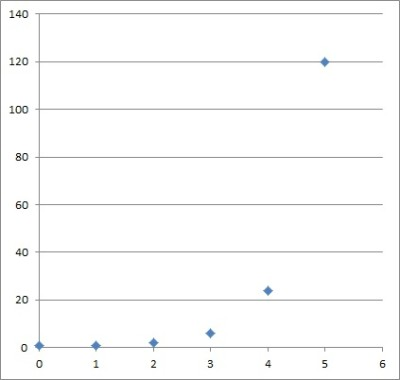# What Is A Factorial? (3 Key Concepts To Learn)

Factorials are useful throughout mathematics: they are found in binomial coefficients, combinatorics, probability distributions, power series expansions (for ex, sin(x), and cos(x)) and the gamma function.

So, what is a factorial?  The factorial of a positive integer n is the product of all integers from 1 to n. The factorial of n is denoted by n! and spoken as “n factorial”. The equation to calculate a factorial is: n! = n*(n-1)*(n-2)*(n-3)*…*3*2*1. A factorial is undefined unless n is a nonnegative integer, and 0! = 1.

Of course, we cannot take the factorial of a decimal, a fraction, or a negative number.  A factorial will always be an integer, since it is a product of integers.

In this article, we will talk about factorials, what they are, and how they are calculated and simplified.  We will also look at the possible inputs and outputs for factorials, along with some examples.

Let’s get started.

## What Is A Factorial?

A factorial is a mathematical operation that takes an input (a nonnegative integer n) and gives an output (a positive integer n!).The factorial of n is the product of all positive integers from 1 to n. So, 5 factorial or 5! is 5*4*3*2*1 = 120.

For a given input n, there is a single output n!.  So, we can think of factorial as a function, defined by:

• f(n) = n! for nonnegative integers n

By convention, we have f(0) = 0! = 1 for n = 0.  For any positive integer n, n! is the product of the n integers from 1 to n.

In other words:

• f(n) = n! = n*(n-1)*(n-2)*…*3*2*1

A factorial function grows faster than an exponential function, as Stirling’s approximation shows.A graph showing the values of the first 6 values of f(n) = n!, from n = 0 to n = 6. This function grows faster than an exponential function.

### How Is Factorial Calculated?

A factorial is often calculated by “brute force” – that is, by multiplying together all of the numbers from 1 to n.

However, we can also use a computer to do it.  For example, here is computer code to calculate a factorial using a function that contains a for loop:

``````int factorial(int n){
int product = 1;
for(i = 1, i <= n, i++){
product = product*i;
}
return product;
}``````

We could also use a recursive function to calculate a factorial:

``````int factorial(int n){
if(n < 0){
return error;
else if(n == 0 || n ==1){
return 1;
}else{
return n*factorial(n – 1);
}
}``````

A table of factorials, like the one below, can help you to calculate faster.  Memorizing the table for the first 5 or 6 will speed things up as well.

### What Is The Factorial Of 0?

The factorial of 0 is 1.  This is by convention, but we can also offer a proof of why this should be true, as follows.

First, we write out table of several factorials:

To get from one row in the table to the next, we divide by n.  For example, to go from 5! To 4!, we divide by 5 (120 / 5 = 24).  Similarly:

• To go from 4! to 3!, we divide by 4 (24 / 6 = 4).
• To go from 3! to 2!, we divide by 3 (6 / 3 = 2).
• To go from 2! to 1!, we divide by 2 (2 / 2 = 1).

So, to go from 1! To 0!, we divide by 1.  Since 1 / 1 = 1, we can see that 0! = 1.

### Can Factorials Be Negative?

Factorials cannot be negative.

The result (or output) of a factorial will always be a positive integer, since it is a product of positive integers from 1 to n, where n is positive (or for n = 0, 0! = 1).

The input for a factorial cannot be negative either.  The input for a factorial must be nonnegative, since n! is only defined for n >= 0.

(You can learn about the gamma function here –  it is defined by an integral and extends the factorial function to the complex numbers).

### Can Factorials Be Decimals?

Factorials cannot be decimals.

As mentioned earlier, the result (or output) of a factorial will always be a positive integer, since it is a product of positive integers from 1 to n, where n is positive (or for n = 0, 0! = 1).

(See the gamma function mentioned above).

### Can You Do A Factorial Of A Decimal?

You cannot do a factorial of a decimal.

The input for a factorial must be a positive integer, which means no decimals are allowed.

(See the gamma function mentioned above).

### Can You Do A Factorial Of A Fraction?

You cannot do a factorial of a fraction.

The input for a factorial must be a positive integer, which means no fractions are allowed.

(See the gamma function mentioned above).

### Can Factorials Cancel?

Factorials can cancel if we have a fraction with factorials in both the numerator and denominator.

By definition of a factorial, we can write n! in many different ways.  For example, when n is large enough, we can write n! = n*(n-1)!, since:

• n! = n*(n-1)*(n-2)*…*3*2*1
• n! = n*[(n-1)*(n-2)*…*3*2*1]  [separate the last n-1 factors into a separate product]
• n! = n*(n-1)!  [since (n-1)!  = [(n-1)*(n-2)*…*3*2*1]

Similarly, we can also write:

• n! = n*(n-1)*(n-2)!
• n! = n*(n-1)*(n-2)*(n-3)!
• n! = n*(n-1)*(n-2)*(n-3)*(n-4)!
• …etc.

Let’s look at some examples of how factorials cancel.

#### Example 1: Cancel Factorials

Let’s say we have the fraction 5!/3!.

We can rewrite 5! As 5*4*3!.

So, we can simplify the fraction as:

• 5! / 3!
• =5*4*3! / 3!  [rewrite 5! In terms of 3!]
• =5*4  [cancel 3! in numerator and denominator]
• =20

We can also verify this by using 5! = 120 and 3! = 6 to get 120/6 = 20.  However, for large values of n, it is easier to use cancellation, as you can see in the next example.

#### Example 2: Cancel Factorials

Let’s say we have the fraction 500!/498!

We can rewrite 500 as 500*499*498!

So, we can simplify the fraction as:

• 500! / 498!
• =500*499*498! / 498!  [rewrite 500! In terms of 498!]
• =500*499  [cancel 498! in numerator and denominator]
• =249,500

#### Example 3: Cancel Factorials

Let’s say we have the fraction 10! / 7!3!

We can rewrite 10! as 10*9*8*7!

So, we can simplify the fraction as:

• 10! / 7!3!
• =10*9*8*7! / 7!3!  [rewrite 10! In terms of 7!]
• =10*9*8 / 3!  [cancel 7! in numerator and denominator]
• =720/6
• =120

We can also simplify factorials when there are variables involved, as the next example shows.

#### Example 4: Cancel Factorials With Variables

Let’s say we have the fraction x!/(x – 3)!

We can rewrite x! as x*(x-1)*(x-2)*(x-3)!

So, we can simplify the fraction as:

• x! / (x-3)!
• = x*(x-1)*(x-2)*(x-3)! / (x-3)!  [rewrite 500! In terms of 498!]
• =x*(x-1)*(x-2)  [cancel (x-3)! in numerator and denominator]
• =x*(x2 – 3x + 2)  [FOIL product of binomials]
• =x3 – 3x2 + 2x  [distribute x through parentheses]

### Can You Distribute A Factorial?

You cannot distribute a factorial in the same way that you can distribute multiplication through parentheses.

For multiplication, it is true that a*(b + c) = a*b + a*c.

However, for factorials:

• (a + b)! is not always equal to a! + b!, and
• (ab)! is not always equal to a!b!

Consider these example as proof:

Take a = 2 and b = 3.  We can calculate:

• (a+b)! = (2+3)! =5! = 5*4*3*2*1 = 120
• a! + b! = 2! + 3! = 2 + 6 = 8

Since 120 is not equal to 8, we can see that (a+b)! is not equal to a! + b! in all cases.  However, it is true if either a or b is 0.

Taking a = 2 and b = 3 again, we can calculate:

• (ab)! = (2*3)! = 6! = 6*5*4*3*2*1 = 720
• a!b! = 2!3! = 4*6 = 24

Since 720 is not equal to 24, we can see that (ab)! is not equal to a!b! in all cases.  However, it is true if either a or b is 1.

### Can You Take The Derivative Of A Factorial?

For the factorial function f(n) = n! defined for nonnegative integers n, we cannot take the derivative.  The reason is that the function is not continuous on the real numbers.

However, we can take the derivative of the gamma function, which is a way to extend the factorial function to complex numbers.

## Conclusion

Now you know what a factorial is, along with the possible inputs and outputs for this important mathematical operation.